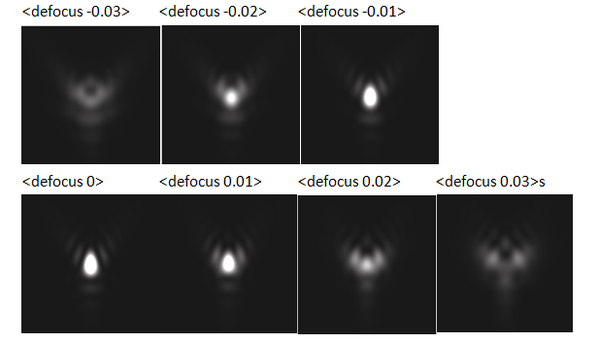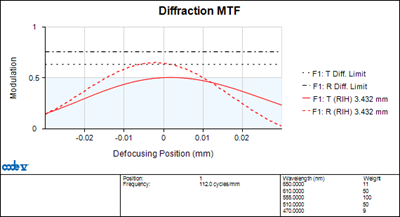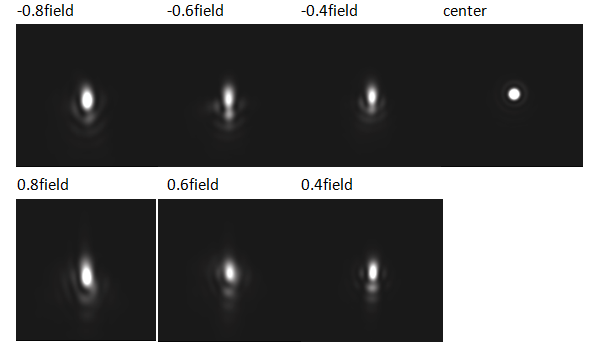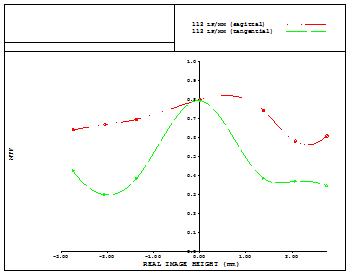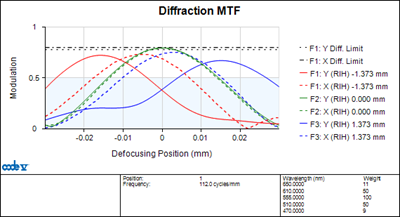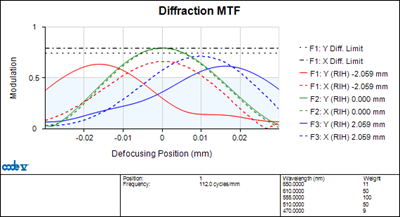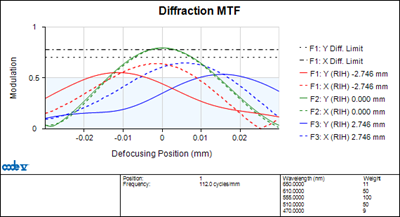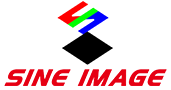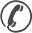全国服务热线：400-666-2522

# 镜头MTF测试解析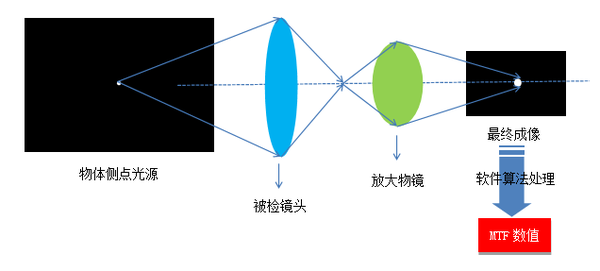## 测试光源

MTF 测试中常用三种发光体：点，狭缝，刃边。以下将逐一介绍如何用这三种光源。

## 点光源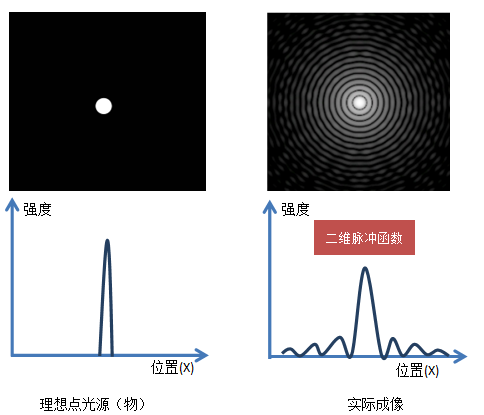g(x, y) = f(x, y)*h(x, y)

G(fx, fy) = F(fx, fy)H(fx, fy)

OTF(fx, fy) = |H(fx, fy)|expφ(fx, fy)

## 狭缝光源

f(x,y) = δ(x)l(y)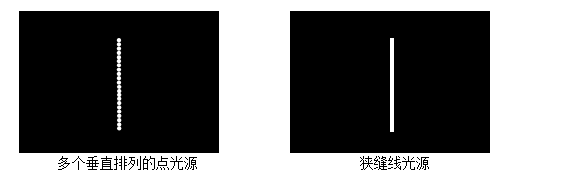g(x, y) = LSF(x) = f(x, y)*h(x, y) = [δ(x)l(y)]*PSF(x, y)

## 刃边光源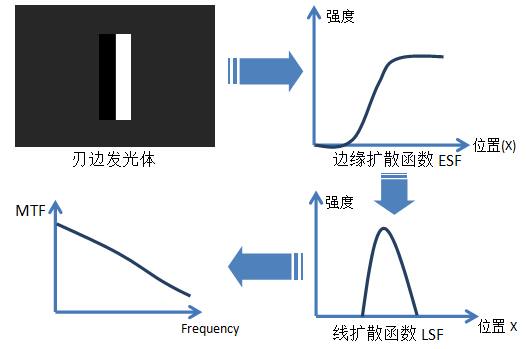## MTF分析实例

a) 搜索到中心视场的点像，记录下此时defocus位置P0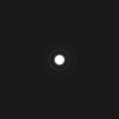b) 从P0 - 0.03位置开始摄取点像并计算MTF，假定step为0.01，测到P0 + 0.03。 （如果焦深很大，则P0两侧的defocus测定范围也要相应的扩大）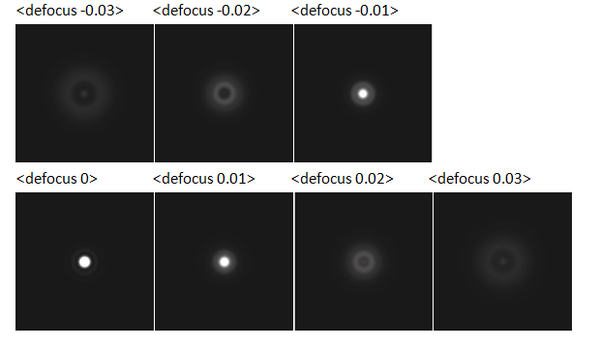7个defocus位置的MTF近似绘制MTF through focus曲线，设备软件计算出peak位置，并移动物镜对焦在peak位置上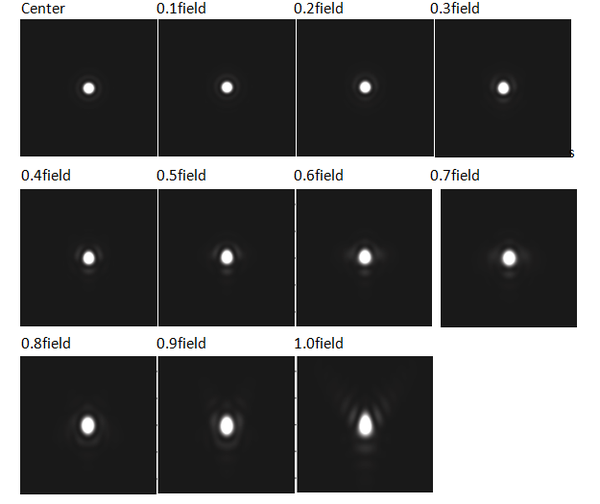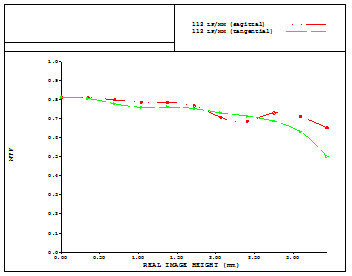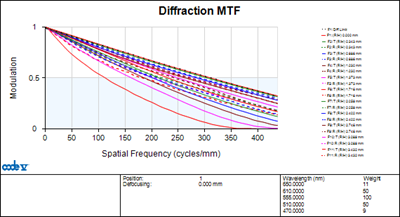1.0field T.S example